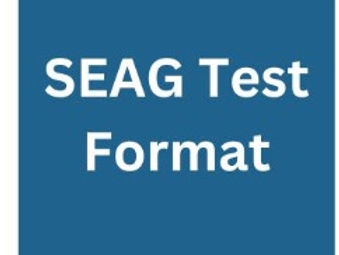top of page

# Format of SEAG Entrance TestWhat is the format of the new SEAG Transfer Test?

The new SEAG Entrance Assessment will consist of two papers. Paper 1 will be taken on 11th November 2023 and Paper 2 on 25th November 2023. Both papers assess English (or Gaeilge) and Mathematics and both have an identical format consisting of three Sections:

The first Section is the Practice Test Section.

It has 5 English (or Gaeilge questions) followed by 5 Maths questions. These questions are designed to help pupils settle before the Main Test begins. The Practice Test is not assessed.

The second Section is the English (or Gaeilge) Main Test.

It has 28 questions(Q1-Q28).

There is a “Punctuation Exercise” containing 5 multiple choice questions, a “Grammar Exercise” also with 5 multiple choice questions and a “Spelling Exercise” with 5 multiple choice questions (Q1-Q15).

This is followed by an “English Comprehension”. Pupils carefully read a passage and are then asked 13 questions; 7 of these are multiple choice (Q16-Q22). The final 6 questions (Q23-Q28) are described as “free response”; the pupil writes the answer to each in the space provided in the Answer Sheet.

The third Section is the Maths Main Test.

It also has 28 questions (Q29-Q56).

The first 22 Maths questions (Q29-Q50) are multiple choice questions. The final 6 Maths questions (Q51-Q56) are described as “free response”. For each of these questions the pupil works out the correct answer and writes the answer in the space provided in the Answer Sheet.

• Pupils will have 60 minutes to answer the 56 questions.

• Each of the multiple choice questions has 5 possible answers.

bottom of page